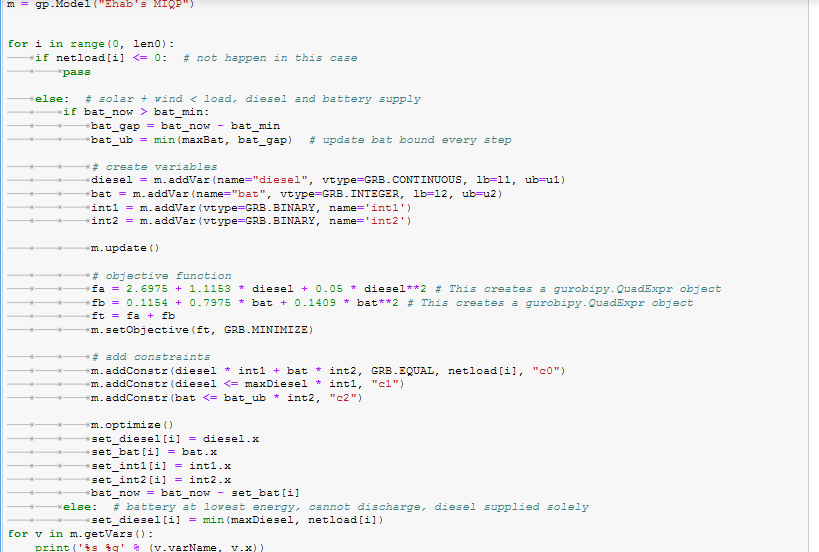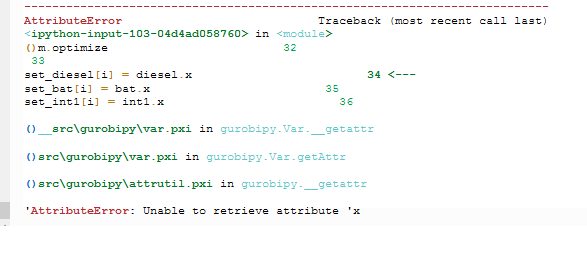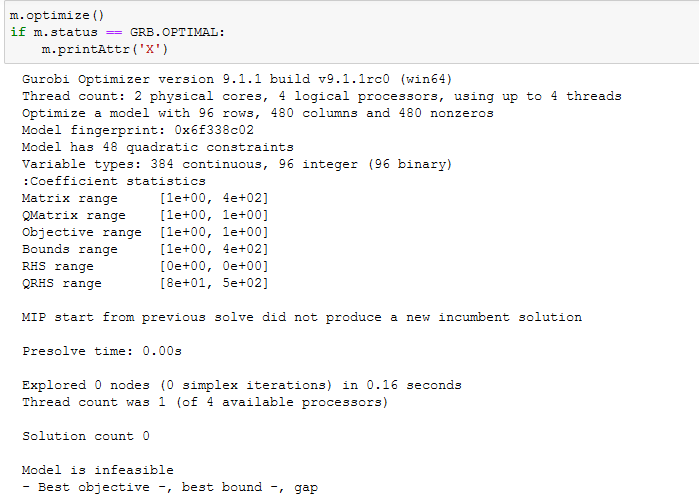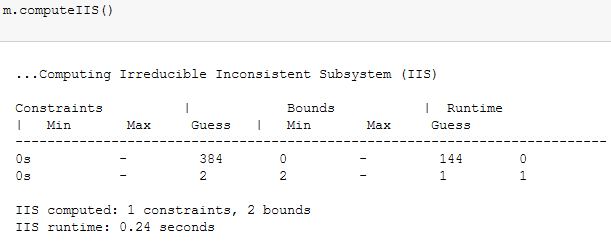•Gurobi Staff

To give you a feel for how you could implement your example in Gurobi, I adapted our qcp.py example to your post:

# This is adapted from the example in https://www.gurobi.com/documentation/9.1/examples/qcp_py.html#subsubsection:qcp.pyimport gurobipy as gpfrom gurobipy import GRB# Create a new modelm = gp.Model("Ehab's MIQP")# Create variables# From your post:#    l ≤ X1 ≤ u #    l ≤ X2 ≤ u# For illustrative purposes, I made X1 continuous and X2 integerl = 0u = 5X1 = m.addVar(name="X1", vtype=GRB.CONTINUOUS, lb=l, ub=u) X2 = m.addVar(name="X2", vtype=GRB.INTEGER, lb=l, ub=u)# Set objective:# From your post:#    ft=fa+fb #    fa = ai + bi * X1 + ci * X1^2#    fb = ai + bi * X2 + ci * X2^2ai = 1bi = 2ci = 3fa = ai + bi * X1 + ci * X1**2 # This creates a gurobipy.QuadExpr objectfb = ai + bi * X2 + ci * X2**2 # This creates a gurobipy.QuadExpr objectft = fa + fbm.setObjective(ft, GRB.MINIMIZE)# Add constraints# From your post:#    A X1+B X2 = b (linear constraints)A = 2B = 4b = 10m.addConstr(A*X1 + B*X2 == b , "c0")m.optimize()for v in m.getVars():    print('%s %g' % (v.varName, v.x))print('Obj: %g' % ft.getValue())

For more information, I recommend you check out the Gurobi Example page and the Python API Details.

•Thanks a lot for the great explanation

•i adapted my problem with code but after running get this error

Can you help me to solve it ?

#A=int1#B=int2#X1 = Diesel#X2 = batl1 = 0l2 = 0 u1 = maxDieselu2 = bat_ubm = gp.Model("Ehab's MIQP")for i in range(0, len0):	if netload[i] <= 0:  # not happen in this case		pass	else:  # solar + wind < load, diesel and battery supply		if bat_now > bat_min:			bat_gap = bat_now - bat_min			bat_ub = min(maxBat, bat_gap)  # update bat bound every step			# create variables			diesel = m.addVar(name="diesel", vtype=GRB.CONTINUOUS, lb=l1, ub=u1) 			bat = m.addVar(name="bat", vtype=GRB.INTEGER, lb=l2, ub=u2)			int1 = m.addVar(vtype=GRB.BINARY, name='int1')			int2 = m.addVar(vtype=GRB.BINARY, name='int2')			m.update()			# objective function			fa = 2.6975 + 1.1153 * diesel + 0.05 * diesel**2 # This creates a gurobipy.QuadExpr object			fb = 0.1154 + 0.7975 * bat + 0.1409 * bat**2 # This creates a gurobipy.QuadExpr object			ft = fa + fb			m.setObjective(ft, GRB.MINIMIZE)			# add constraints			m.addConstr(diesel * int1 + bat * int2, GRB.EQUAL, netload[i], "c0")			m.addConstr(diesel <= maxDiesel * int1, "c1")			m.addConstr(bat <= bat_ub * int2, "c2")			m.optimize()			set_diesel[i] = diesel.x			set_bat[i] = bat.x			set_int1[i] = int1.x			set_int2[i] = int2.x			bat_now = bat_now - set_bat[i]		else:  # battery at lowest energy, cannot discharge, diesel supplied solely			set_diesel[i] = min(maxDiesel, netload[i])for v in m.getVars():    print('%s %g' % (v.varName, v.x))print('Obj: %g' % ft.getValue())••Gurobi Staff

Hi Ehab,

It looks like you are unable to retrieve the solution value for your variables ($$\texttt{variable.X}$$) because the solution is infeasible.  You are smart to add a check of the $$\texttt{m.status}$$ value before trying to retrieve this attribute.

If you want some advice on dealing with infeasibility, I recommend checking this out: How do I determine why my model is infeasible?

•I actually read the article, but I couldn't find the steps to solve the problem

•Gurobi Staff

Hi Ehab,

You are unable to retrieve solution values because the model is infeasible.  You can see this in the log file:

...Model is infeasible- Best objective, -, best bound -, gap

In order to get solution values, you will need to address why your model is infeasible.  I simply included the article to help you determine why your model is infeasible.